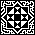# Maths Puzzles

I need to do a little more work here, adding some more puzzles and writing up full solutions to those that don't have them. Try your hand at what's here if you like, but there's no prizes other than the knowledge of your success. Where there's a link to a solution, there's also a hint if you just need a helpful tip to get you started. Most of these are either traditional puzzles, or extensions to other people's puzzles. A few are original.

• What is the expanded value of (a - x)(b - x)(c - x)...(z - x)? [SOLUTION]

• In this game there are three pots, one containing 3 counters, one containing 5 counters, and one containing 7 counters. One their turn a player takes as many counters as they like from one of the pots only (minimum 1 counter). The player to take the last counter loses. In a two player game, what is the winning strategy? [SOLUTION]

• A perfect circle is contained entirely within a perfect quarter circle, both of which lie on the same two-dimensional simple Euclidean plane. What is the maximum amount of the area of the quarter circle that the circle can fill?

• Provide a geometric proof of the formula tan2θ = (2tanθ)/(tan²θ + 1).

• Prove that tan50° + tan60° + tan70° = tan80°. [SOLUTION]

• What is the first derivative of xx and hence of f(x)g(x)? [SOLUTION]

• A point is chosen within a rectangle. Upon drawing lines from the point to each corner of the rectangle, it is discovered that one of the lines is of length 2, one of length 3, and another of length 4. What is the length of the fourth line? And what are the possible lengths of the rectangle's sides?

• You have 12 coins, 11 of which are the same, but one of which is a fake. The fake can be detected because it has a different weight to the other 11, and you have a set of scales to help you work out which it is. But the scales will tell you only which side is heavier or lighter, you don't know whether the fake is too heavy or too light, and you have only three uses of the scales to come to your conclusion. Can you say which is the fake coin, and whether it is too heavy or too light? [SOLUTION]

• Felix played a game where he rolled five dice, six times. In each of these six turns, he would select a number (1 to 6) and score the total value of that number on the dice. So a roll of 2,2,6,6,6 would score him 4 in the 2's, 18 in the 6's, and 0 anywhere else, although Felix never scored 0 on any turn. He played this game a total of five times, scoring the same total in each game, but he didn't score the same value across a row of his scoring grid. In more than one of his games, he rolled five of more than one number, but these games were not consecutive. In any game where Felix rolled five of an odd number, he didn't roll an odd number of any other odd number. For every game x, the number of 6's rolled was 6-x and for all but one of these games, he rolled five x's. Complete his scoring grid. [SOLUTION]

G   A   M   E
| 1 | 2 | 3 | 4 | 5 |
---+---+---+---+---+---+
1's|   |   |   |   |   |
S ---+---+---+---+---+---+
2's|   |   |   |   |   |
C ---+---+---+---+---+---+
3's|   |   |   |   |   |
O ---+---+---+---+---+---+
4's|   |   |   |   |   |
R ---+---+---+---+---+---+
5's|   |   |   |   |   |
E ---+---+---+---+---+---+
6's|   |   |   |   |   |
---+---+---+---+---+---+